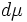Total scalar curvature

This article defines a notion of curvature for a differential manifold equipped with a Riemannian metric

This article defines a scalar value (viz, a real number) associated with a Riemannian manifold. This real number depends both on the underlying differential manifold and the Riemannian metric

Definition

Given data

A compact connected differential manifold$M$ with a Riemannian metric$g$.

Definition part

The total scalar curvature of$M$ is defined as the integral over the volume of the scalar curvature over the manifold. That is, if$R$ denotes the scalar curvature and$d\mu$ the volume element, we have that:$r = \int R d\mu$

Here, the volume element$d\mu$ is the natural choice of volume-element arising from the Riemannian metric.

Note that if we divide by the total volume, we get the average scalar curvature.

Further information: average scalar curvature